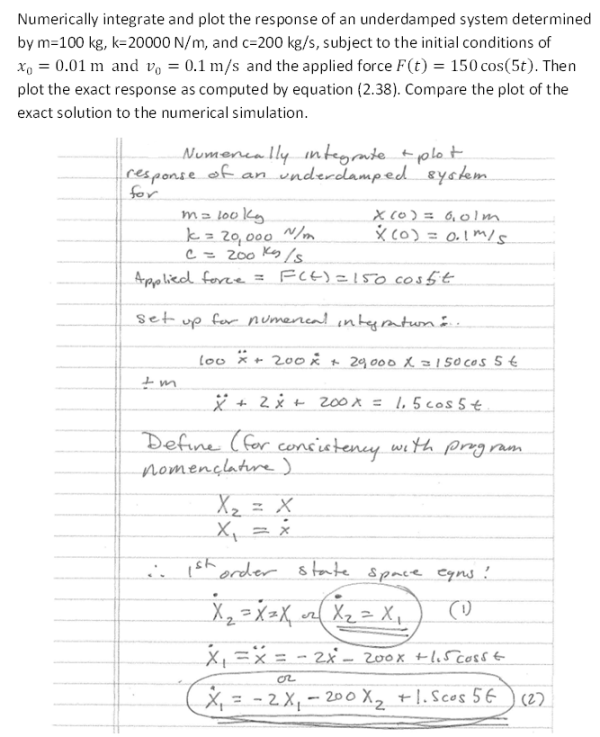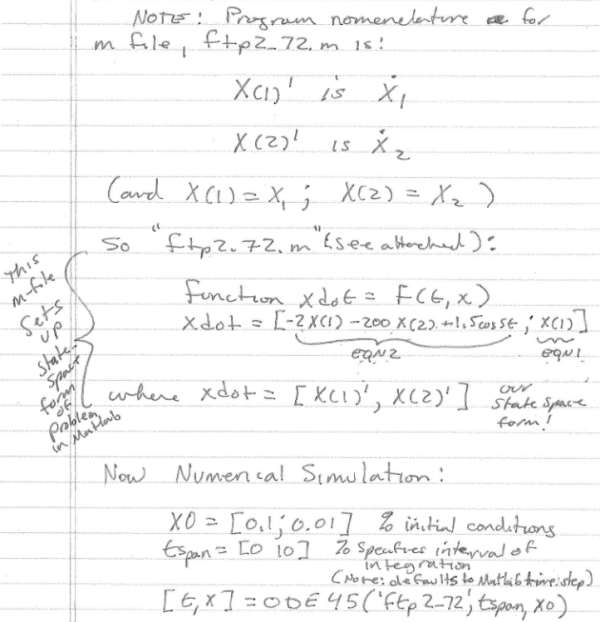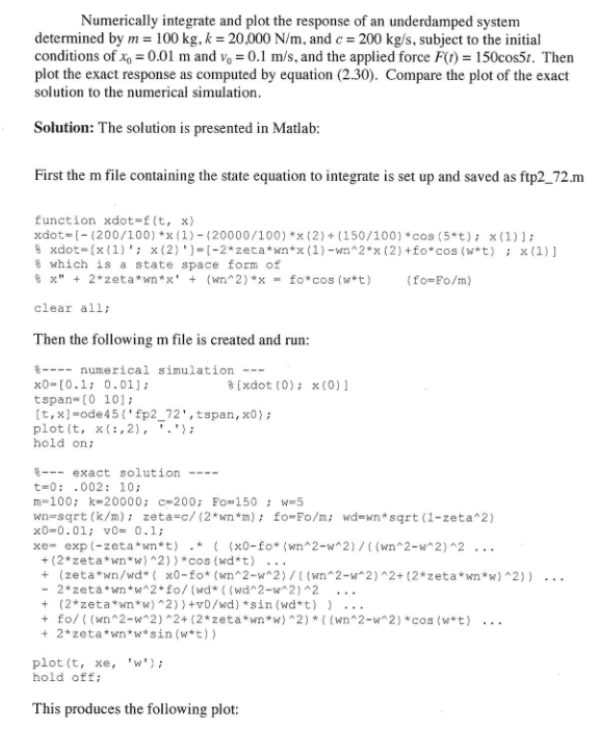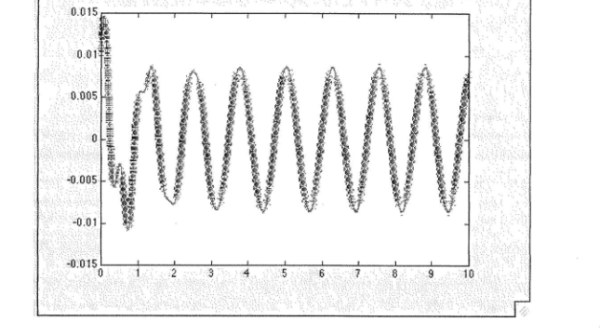Numerically integrate and plot the response of an underdamped system determined by m=100 kg, k=20000 N/m, and c=200 kg/s, subject to the initial conditions of x0=0.01 m and v0=0.1 m/s and the applied force F(t)=150cos(5t). Then plot the exact response as computed by the equation. Compare the plot of the exact solution to the numerical simulation.Numerically integrate and plot the response of an underdamped system determined by m=100 kg, k=20000 N/m, and c=200 kg/s, subject to the initial conditions of x0=0.01 m and v0=0.1 m/s and the applied force F(t)=150cos(5t). Then plot the exact response as computed by the equation. Compare the plot of the exact solution to the numerical simulation.

Vibrations Page 1 vibrations vibrations vibrations vibrations vibrations vibrations vibrations Vibrations Page 2